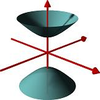#### You may also like### A Close Match

Can you massage the parameters of these curves to make them match as closely as possible?### The Right Volume

Can you rotate a curve to make a volume of 1?### The Jabber-notty

Can you invert this confusing sentence from Lewis Carrol?

# Prime Counter

##### Age 16 to 18Challenge Level

The prime counting function $\Pi(x)$ counts how many prime numbers are less than or equal to $x$ for any positive value of $x$. Since the primes start $2, 3, 5, 7, 11, 13, \dots$ we therefore have, for example, $\Pi(11) = 5$ and $\Pi(8) = 4$.

It is believed by mathematicians that $\frac{x}{\ln(x)}$ is a good approximation to $\Pi(x)$. It is believed to get progressively better as $x$ increases to very, very large numbers. How well does it work for lower values of $x$ (up to the 100,000th prime)

Explore the percentage accuracy of this approximation.

Use a few sensible values / choice of axes to try to create a useful graphical representation of $\ln(\Pi(x))$ against $\ln(x)$ for $x$ taking values up to about a million. Use your curve to try to predict $\Pi(x)$ for a few values of $x$ away from your data points. How close are your estimates for whole number multiples of $100,000$?

Use your judgement to try to extrapolate your curve to make approximations as to
$$\Pi(10^7)\quad\quad \Pi(10^8)\quad\quad \Pi(10^9)$$

Did you know ... ?

Although prime numbers are distributed with some large-scale regularity across the natural numbers, as this problem indicates, there is no known process which generates the prime numbers.